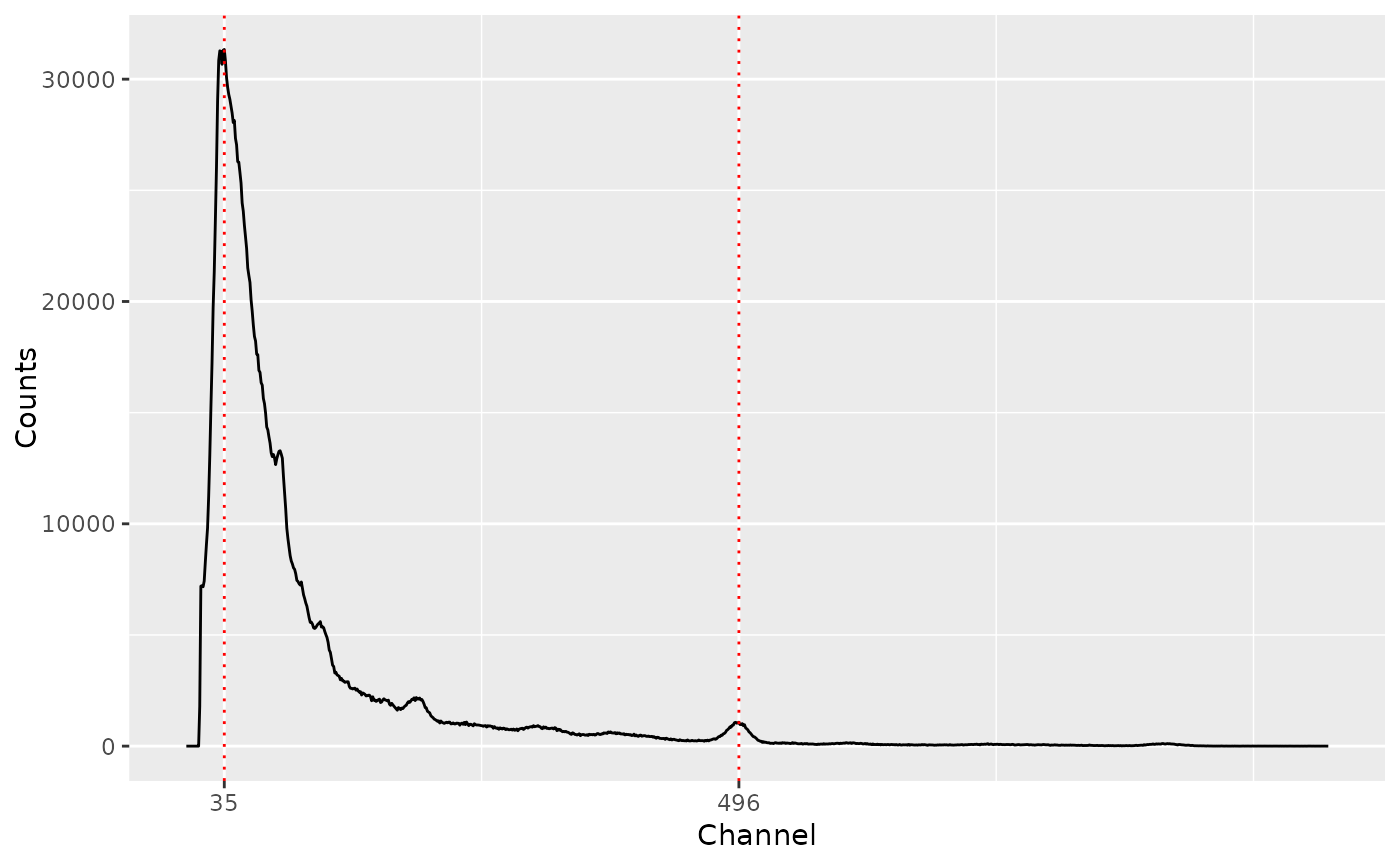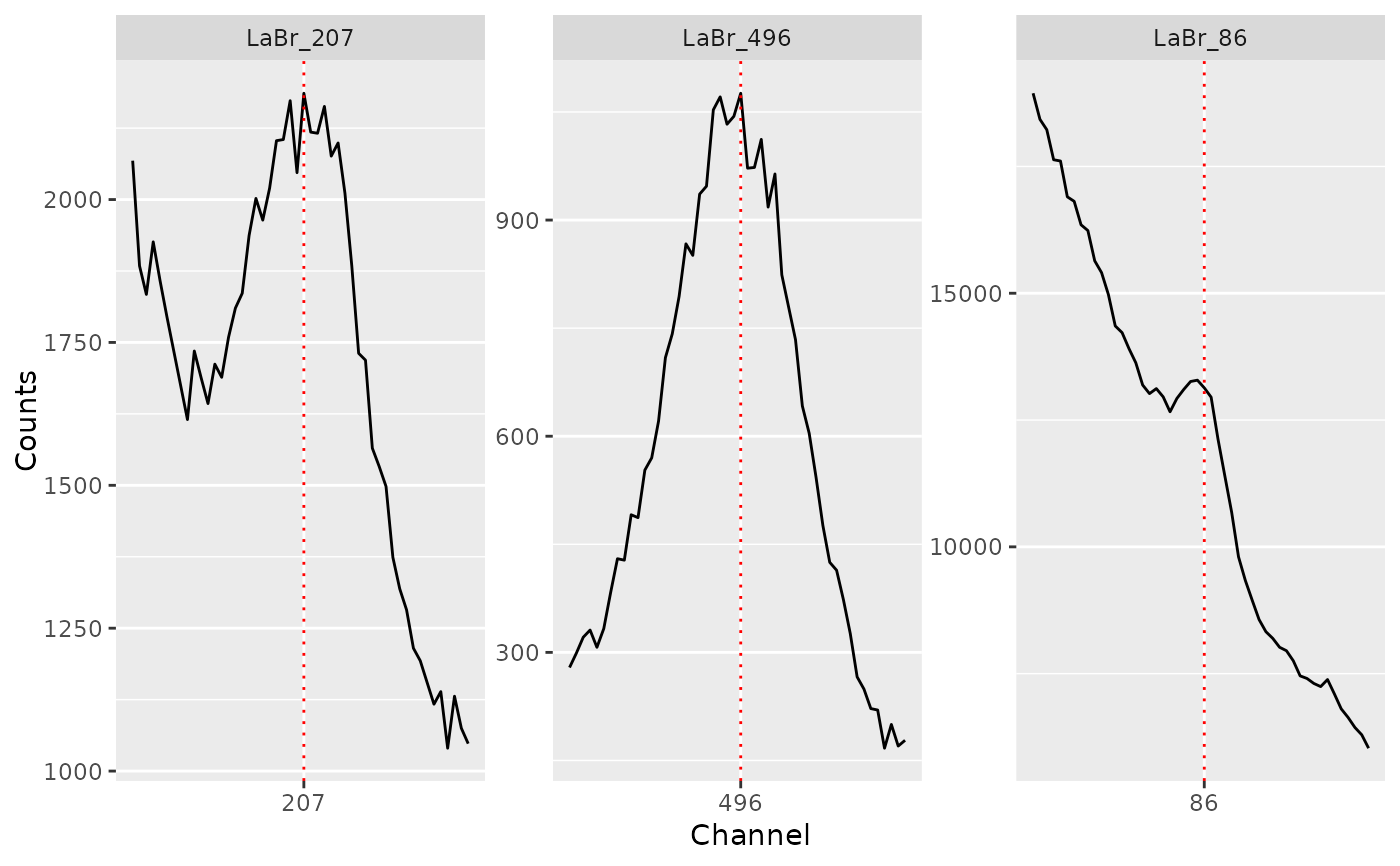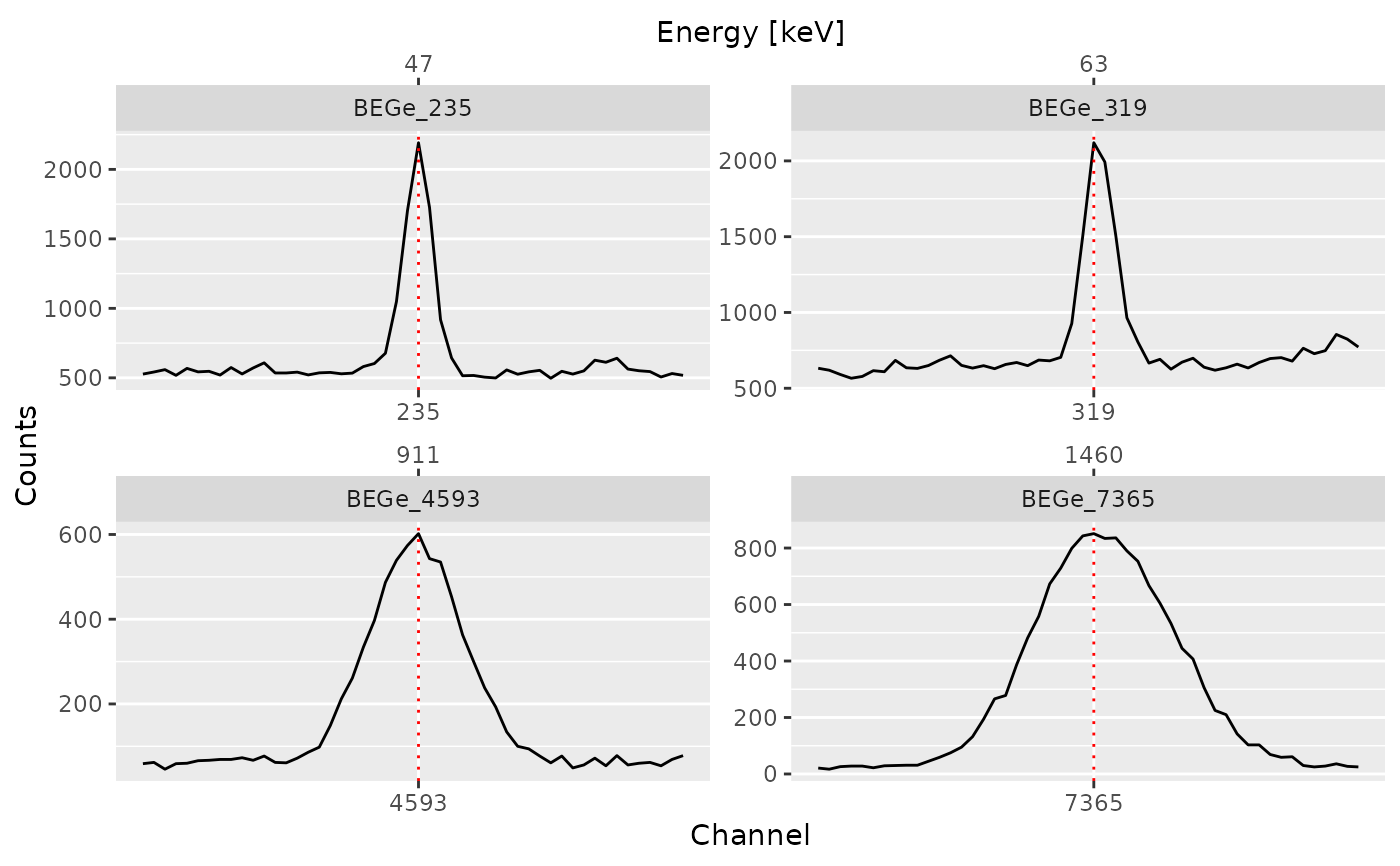Finds local maxima in sequential data.

## Usage

peaks_find(object, ...)

# S4 method for GammaSpectrum
peaks_find(object, method = c("MAD"), SNR = 2, span = NULL, ...)

## Arguments

object

A GammaSpectrum object.

...

Extra parameters to be passed to internal methods.

method

A character string specifying the method to be used for background noise estimation (see below).

SNR

An integer giving the signal-to-noise-ratio for peak detection (see below).

span

An integer giving the half window size (in number of channels). If NULL, 5\ window size.

## Value

A PeakPosition object.

## Details

A local maximum has to be the highest one in the given window and has to be higher than $$SNR \times noise$$ to be recognized as peak.

The following methods are available for noise estimation:

MAD

Median Absolute Deviation.

Other signal processing: baseline, peaks_search(), signal_integrate(), signal_slice(), signal_split(), signal_stabilize(), smooth()

N. Frerebeau

## Examples

## Import a LaBr spectrum
LaBr_file <- system.file("extdata/LaBr.TKA", package = "gamma")

## Find peaks by channel
(LaBr_pks <- peaks_find(LaBr_spc)) # Ugly
#> 2 peaks were detected.
plot(LaBr_spc, LaBr_pks)## Search peaks by channel
(LaBr_pks <- peaks_search(LaBr_spc, index = c(86L, 207L, 496L), span = 7))
#> 3 peaks were detected.
plot(LaBr_spc, LaBr_pks, split = TRUE)## Import a BEGe spectrum
BEGe_file <- system.file("extdata/BEGe.CNF", package = "gamma")